### 第一章 线性方程组

#### 1.1 线性方程组 高斯消元法与矩阵

• 当 $b_i$ 全为 0 时，称为齐次线性方程组；当 $b_i$ 不全为 0 时，称为非齐次线性方程组。
• 包含相同变量的两个方程组有相同解，则它们是等价的。
• 线性方程组的初等变换:
• 交换方程组两个方程的顺序（矩阵行的顺序）；
• 在一个方程两端乘以一个非零的常数（矩阵行乘常数）；
• 一个方程常数倍加在另一个方程上。

• 增广矩阵和系数矩阵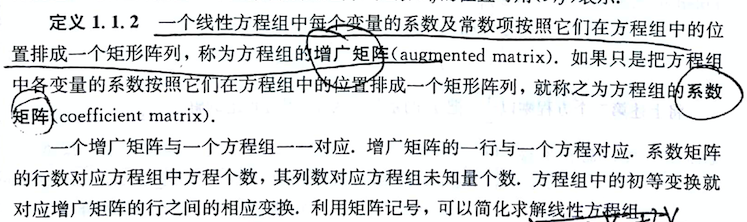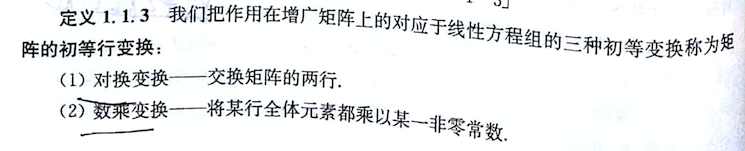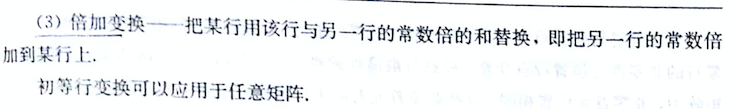• 两个矩阵可以通过初等行变换进行转换，则两个矩阵等价。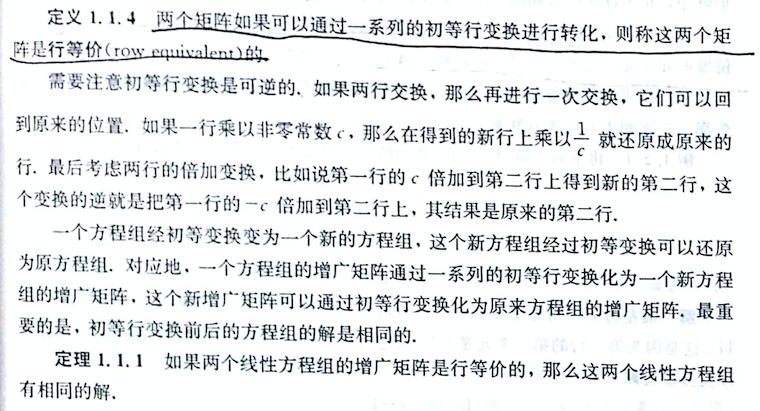#### 1.2 行化简和阶梯型矩阵 解的存在性和唯一性

• 阶梯矩阵（首元位置唯一）和行最简形矩阵（唯一）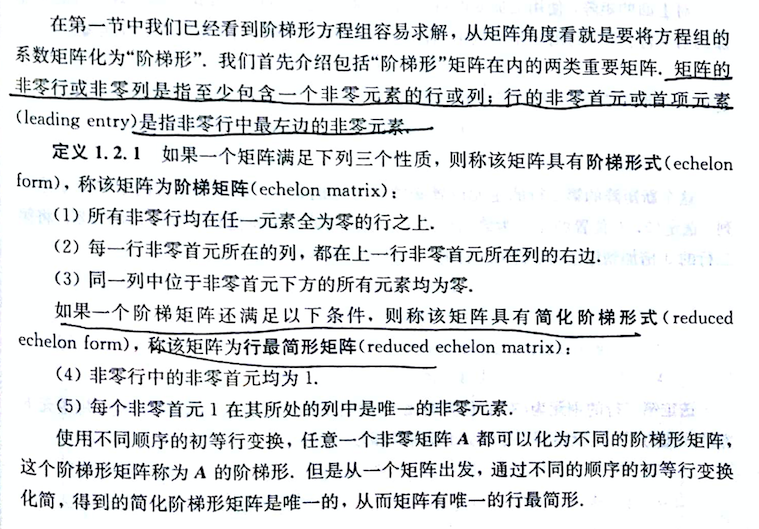• 主元和主元列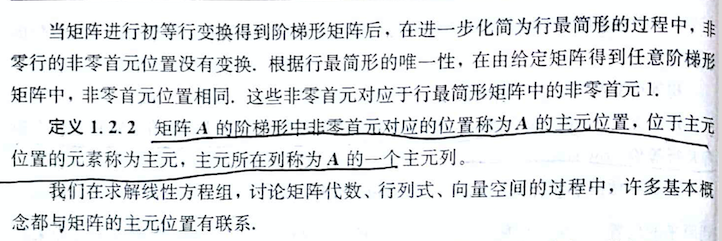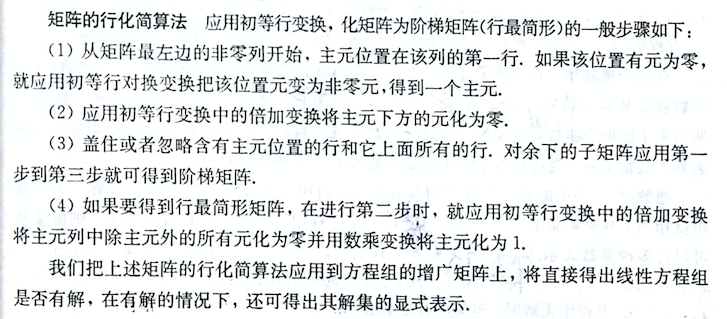• 主元的变量称为基本变量，非主元称为自由变量。自由变量由基本变量确定。主元列数（以后是矩阵的秩）小于变量个数，有无穷多解。$m\times n$ 的矩阵，当 $m<n$ 时，齐次线性方程组一定有非零解（无穷多解）。

• 增广矩阵存在 $[0,0,\cdots,b]$ 无解。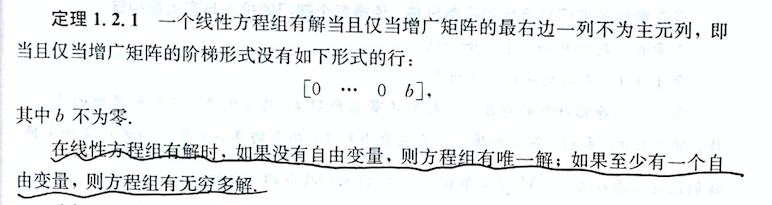$$\begin{bmatrix} 1 & 1 & 1 & 1 & 1 & 1\\ 0 & 0 & 1 & 1 & 2 & 1\\ 0 & 0 & 0 & 0 & 1 & 3\\ 0 & 0 & 0 & 0 & 0 & 3\\ 0 & 0 & 0 & 0 & 0 & 0 \end{bmatrix}$$

• 初等行变换求解矩阵### 第二章 矩阵代数

#### 2.1 矩阵与矩阵运算

• 矩阵 $A$ 和 $B$ 的维度相同，则称为同型矩阵。两个矩阵对应元素相等，则 $A=B$。

• 矩阵的乘法，例如$AB$，$A$ 的列数必须等于 $B$ 的行数，表示为 $A_{m\times n}B_{n\times s}$

• 矩阵的乘法不满足 交换律，即 $AB\ne BA$，除此之外满足交换律、结合律等。

• 矩阵乘法示例:

• 行向量乘以列向量得到一个标量（数），这种乘法称为形式标量积、数量级（scalar product）或内积。 $$AX=\left[ \begin{array}{ccc} a_1\ a_2\ \cdots\ a_n \end{array} \right]\left[ \begin{array}{ccc} x_1\\x_2\\\vdots\\x_n \end{array} \right ]=a_1x_1+a_2x_2+\cdots+a_nx_n$$

• 非对角线上元素全为 0 的方阵称为对角矩阵。

• 每一个元素都为 0 的矩阵称为零矩阵，即为 $0$ 或 $0_{m\times n}$。

• n 维行向量（$1\times n$）和 n 维列向量（$n\times 1$）。

• $A_kA_l=A_{k+l},(A_k)_l=A_{kl}$，但 $(AB)_k \ne A_kB_k$ 因为不满足交换律。

• 矩阵的多项式。

#### 2.2 一些特殊矩阵及其运算

• 矩阵的逆
• 矩阵的三种初等变换可以通过左乘一系列初等矩阵获得化简单位矩阵 $E$ :

$$(P_kP_{k-1}\cdots P_1)A=E$$ $$P_kP_{k-1}\cdots P_1=A^{-1}$$

• 矩阵的转置: 矩阵的行变为矩阵的列。$A$ 的转置矩阵，记为 $A^T$。满足的性质包括:

• 对称矩阵，$a_{ij}=a_{ji}$，即 $A^T=A$，按对角线对称。

• 反对称矩阵，主对角线为 0，主对角线两侧反号，即 $A^T=-A$。

两个对称阵之和为对称阵，两个反对称阵之和为反对称阵。

$BB^T,A=B+B^T$ 为对称阵，$A=B-B^T$ 为反对称阵。

比较重要的性质是任意矩阵都可以表示为两个对称阵和反对称阵之和:

例如矩阵 $C$，有矩阵 $A=C+C^T,B=C-C^T$，则$C=\frac{A+B}{2}$。

• 正交矩阵: $A^T=A_{-1}$，满足每一行(列)的平方和为 1，任意两个不同行(列)的数量积(内积)为 0。$A,B$ 为正交阵，则 $AB(BA)$ 也为正交阵，但 $A+B$ 不一定为正交阵。

• 分块矩阵则是按照行和列对矩阵分块，运算和矩阵类似，但是需要同型矩阵。例如 $A$ 按列分块为 $[A_1, A_2, \cdots A_n]$。